# Cross-correlation of Okhotsk 2013 late coda¶

## Core phases constructed from cross-correlations of 2013 Okhotsk earthquake reverberations confirm directionality of late coda waves¶

This notebook demonstrates data analysis for a poster presented at a conference in 2016. The analysis is done with ObsPy and uses the HDF5 read/write plugin obspyh5.

If you want to run this notebook locally, have a look at the repository readme. Dependencies of this notebook are ObsPy, h5py, obspyh5, and tqdm.

Reference: Tom Eulenfeld (2016), Core phases constructed from cross-correlations of 2013 Okhotsk earthquake reverberations confirm directionality of late coda waves, doi: 10.6084/m9.figshare.2074702.

## Introduction¶

Ambient noise can be used to construct surface waves by cross-correlation of continuous data between two stations. The cross-correlation basically filters all waves traveling from one station to the other. One station can be considered as a virtual source, the other station as virtual receiver.

Because noise sources mostly stimulate surface waves it is difficult to construct body waves from cross-correlation of ambient noise. However, it is possible to use the coda of earthquakes as a "noise" source stimulating body waves, too. Here, we analyze such a coda wave field of a large deep-ruptured earthquake which effectively stimulated body waves traveling through the Earth.

## Hands on...¶

10 hours of data from world-wide broad-band stations starting with the origin time of the 2013 Okhotsk (Mw 8.3) event were obtained from GeoFon with the ObsPy ArcLink client. Data were bandpassed between 0.05 Hz and 0.1 Hz and downsampled to 1 Hz. In the following, a HDF5 file containing the preprocessed data is downloaded. The file is read in with ObsPy and the obspyh5 plugin. Some basic information is plotted.

In :
from obspy import read
import os.path
from tqdm import tqdm
import urllib.request

def hook(t):
last_b = 
def inner(b=1, bsize=1, tsize=None):
if tsize is not None:
t.total = tsize
t.update((b - last_b) * bsize)
last_b = b
return inner

fname = 'okhotsk_data.h5'
url = 'https://github.com/trichter/misc/raw/master/data/'
if not os.path.exists(fname):
with tqdm(unit='B', unit_scale=True, desc=fname) as t:
urllib.request.urlretrieve(url + fname, datadir + fname, reporthook=hook(t))

# Read the three data files into single ObsPy Stream
print(stream, '\n\n\nHeader of first trace:\n', stream.stats)

okhotsk_data.h5: 104MB [00:04, 23.9MB/s]

853 Trace(s) in Stream:

AI.BELA.04.BHZ | 2013-05-24T05:44:48.600000Z - 2013-05-24T15:44:48.600000Z | 1.0 Hz, 36001 samples
...
(851 other traces)
...
TW.YULB..BHZ | 2013-05-24T05:44:48.619500Z - 2013-05-24T15:44:48.619500Z | 1.0 Hz, 36001 samples

[Use "print(Stream.__str__(extended=True))" to print all Traces]

network: AI
station: BELA
location: 04
channel: BHZ
starttime: 2013-05-24T05:44:48.600000Z
endtime: 2013-05-24T15:44:48.600000Z
sampling_rate: 1.0
delta: 1.0
npts: 36001
calib: 1.0
_format: H5
baz: 191.6679106
distance: 156.695105528
elevation: 262.0
latitude: -77.875
longitude: -34.6269


Data was collected from 853 stations with a sampling rate of 1 Hz. The HDF5 format has the advantage that it can store arbitrary header fields. Here, the metadata attached to the traces contains only station coordinates and the distance to the epicenter in degrees. First let us visualize station locations and the earthquake epicenter.

In :
import cartopy.crs as ccrs
import matplotlib.pyplot as plt

def plot_map(cevent, cstations, label=None):
fig = plt.figure(figsize=(10, 6))
ax.coastlines()
pc = ccrs.PlateCarree()
ax.plot(*list(zip(*cstations))[::-1], 'v', mfc='w', mew=1, mec='k', ms=10, transform=pc)
ax.plot(*cevent[::-1], '*', color='C1', ms=30, transform=pc)
if label:
ax.annotate(label, (0.9, 0.9), xycoords='axes fraction',
weight='bold', size='large')

cevent = [54.912, 153.339]  # latitude and longitude of epicenter
cstations = [(tr.stats.latitude, tr.stats.longitude) for tr in stream]
label = '2013 Okhostk earthquake\nMw8.3, 800km depth\naround 850 stations'
plot_map(cevent, cstations, label)
plt.show()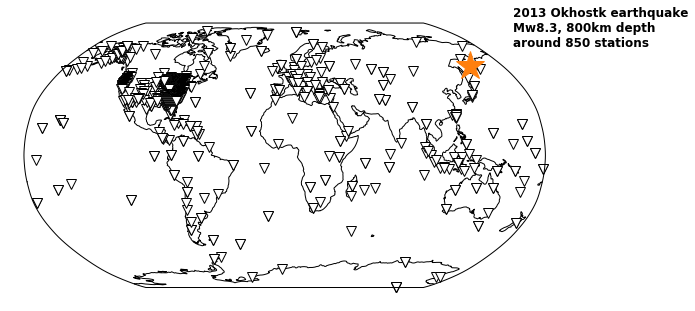How does the data look? To get a first overview we want to produce a classical travel time plot of one hour of data. Because a lot of stations with similar distances to the event exist, waveforms will be stacked first. Here a stack over 0.5° distance bins is created and plotted with a patched version of the ObsPy Stream.plot method with keyword type='section'. The variable wfstack holds the stack of all traces; the distance header contains the mid point of the respective distance bin.

In :
import numpy as np
from obspy import Trace, Stream

def stack_waveforms(stream, ddeg=0.5, fill_all_bins=True):
"""
Stack waveforms in stream (iterator of Traces) in distance bins of ddeg° width.

If fill_all_bins is true a Trace will be created for every distance bin.
If no data is available in a bin it will be filled with zeros.
"""
distbins = np.linspace(0, 180, int(round(180 / ddeg)), endpoint=False)
stack = {}
for tr in stream:
ind = np.digitize(tr.stats.distance, distbins) - 1
if ind not in stack:
header = {'distance': distbins[ind] + 0.5 * ddeg, 'num': 1,
'sampling_rate': tr.stats.sampling_rate,
'starttime': tr.stats.starttime, 'ddeg': ddeg}
else:
tr2 = stack[ind]
tr2.data = tr2.data + tr.data
tr2.stats.num += 1
for tr2 in stack.values():
tr2.data = tr2.data / tr2.stats.num
if fill_all_bins:
nodata = np.zeros(len(tr))
for ind in range(len(distbins)):
if ind not in stack:
header['distance'] = distbins[ind] + 0.5 * ddeg
return Stream(traces=stack.values())

# Slice into stream to stack only the first hour of data
wfstack = stack_waveforms(stream.slice(endtime=stream.stats.starttime + 3600), fill_all_bins=False)

In :
def plot_waveform_stack(stack):
fig = stack.plot(type='section', norm='stream', offset_max=180, handle=True, scale=1)
ax = fig.axes
degs = np.arange(10) * 20
ax.set_xticks(degs / 180. * ax.get_xlim())
ax.set_xticklabels([str(d) for d in degs])
ax.set_xlabel(u'distance to event (°)')
ax.set_yticks(np.arange(7) * 600)
ax.set_ylabel('travel time (s)')

plot_waveform_stack(wfstack)
plt.show()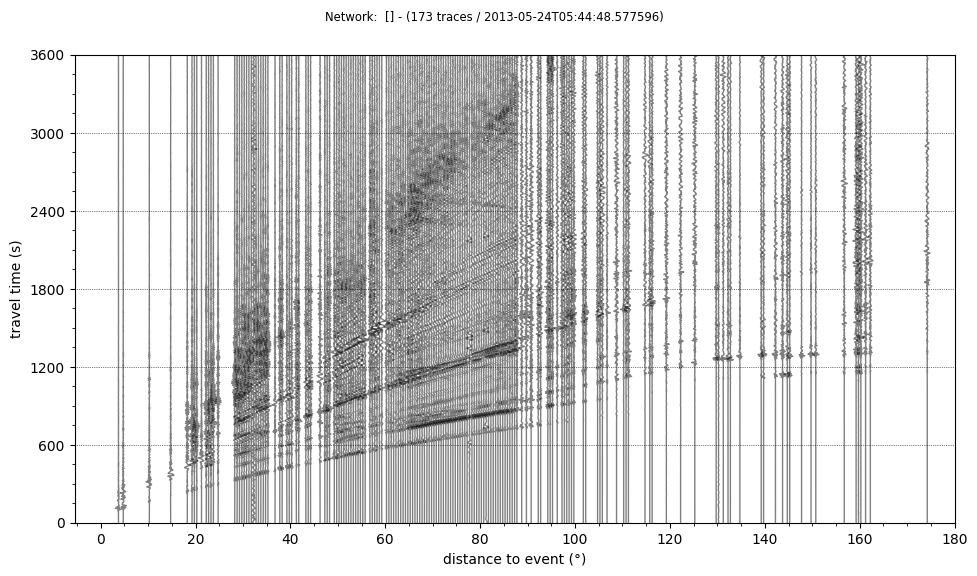OK, this looks good. Visible are surface waves, the P wave, multiples, and core phases like PKP and PKIKP.

## Cross-correlation of the coda and stacking in distance bins¶

But actually we are interested in the coda. So, lets look at an individual waveform of a station located in Germany. The second plot shows a zoomed-in version.

In :
def plot_trace(tr, ylim=None, xcorr=False):
fig = plt.figure(figsize=(17, 1.5))
hours = tr.times() / 3600
if xcorr:
hours = hours - np.max(hours) / 2
ax.plot(hours, tr.data, 'k')
ax.set_xlabel('lag ' * xcorr + 'time (hours)')
#ax.set_yticklabels([])
if ylim:
ax.set_ylim(ylim)

tr = stream.select(station='GRFO')
print(tr.id)
plot_trace(tr)
plt.xlim(0, 10)
plt.show()

IU.GRFO..BHZ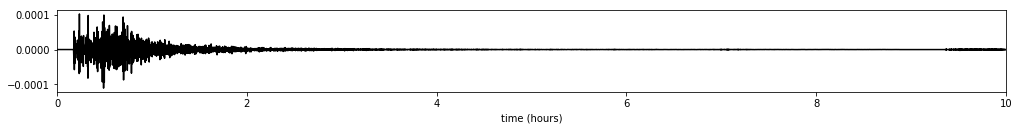In :
plot_trace(tr)
plt.xlim(0, 10)
plt.ylim(-5e-6, 5e-6)  # zoom in to see the late coda
plt.show()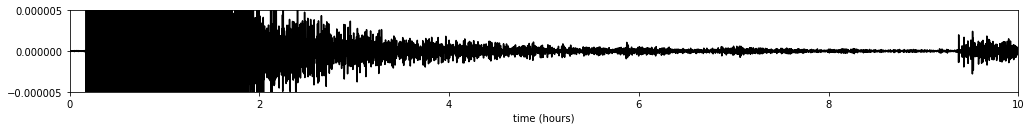We see a nice late coda which we want to use to construct body waves traveling between station pairs. Arrivals of the Mw 6.7 supershear aftershock rupturing around 9 hours after the main shock are visible, too. The time window which will be used for the cross-correlation spans from 10000 to 30000 seconds after the main shock (3.7 to 8.3 hours). We normalize data by dividing through the running mean (of absolute data) over 300 seconds to guarantee equal contribution of early and late parts in the coda. The normalized waveform of the data example above is produced in the following.

In :
def normalize(tr, window=300):
w = np.ones(int(window * tr.stats.sampling_rate), 'd')
smoothed = np.convolve(w / np.sum(w), np.abs(tr.data), mode='same')
tr.data = tr.data / smoothed
return tr

normalized_tr = normalize(tr.copy())
plot_trace(normalized_tr)
plt.xlim(0, 10)
plt.show()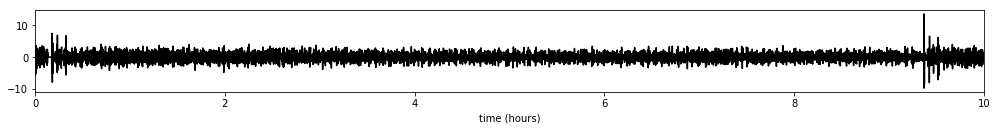Now, we normalize all available data and cut out the late coda.

In :
def prepare(tr, rm_window=300, trim_window=(10000, 30000)):
tr.detrend('linear')
normalize(tr, window=rm_window)
t = tr.stats.starttime
tr.trim(t + trim_window, t + trim_window)

pstream = stream.copy()  # Stream with prepared data
for tr in pstream:
prepare(tr)


Three cross-correlation functions are defined acting on different input parameters:

• xcorr calculates the corss-correlation of two arrays by multiplication in the Fourier domain,
• xcorr_traces correlates two traces and inserts appropriate metadata (e.g. inter-station distance) and
• xcorr_iter correlates all traces inside the passed stream. It returns an iterator, so correlated traces can be processed one after the other. This has the advantage that not all of the large number of cross-correlation have to be held in memory at the same time. (The tqdm function of the same module is used to print a nice progress bar. It can be ignored when going through the code.)

The cross-correlation is calculated and plotted for two selected stations.

In :
from itertools import combinations
from obspy.geodetics import gps2dist_azimuth
from obspy.signal.cross_correlation import correlate

def xcorr_traces(tr1, tr2, maxshift=3600):
n1, s1, l1, c1 = tr1.id.split('.')
n2, s2, l2, c2 = tr2.id.split('.')
sr = tr1.stats.sampling_rate
gps_args = (tr1.stats.latitude, tr1.stats.longitude, tr2.stats.latitude, tr2.stats.longitude)
dist = gps2dist_azimuth(*gps_args) / 1000 / 111.2
xdata = correlate(tr1.data, tr2.data, int(round(maxshift * sr)))
header = {'network': n1, 'station': s1, 'location': n2, 'channel': s2,
'network1': n1, 'station1': s1, 'location1': l1, 'channel1': c1,
'network2': n2, 'station2': s2, 'location2': l2, 'channel2': c2,
'sampling_rate': sr, 'distance': dist}

def xcorr_iter(stream, disable_tqdm=False):
total = (len(stream) * (len(stream) - 1)) // 2
for tr1, tr2 in tqdm(combinations(stream, 2), total=total, disable=disable_tqdm):
yield xcorr_traces(tr1, tr2)

for tr in xcorr_iter(pstream.select(station='GR[FG]?'), disable_tqdm=True):
print('Cross-correlation between stations', tr.id)
plot_trace(tr, xcorr=True)
plt.show()

Cross-correlation between stations CU.GRGR.IU.GRFO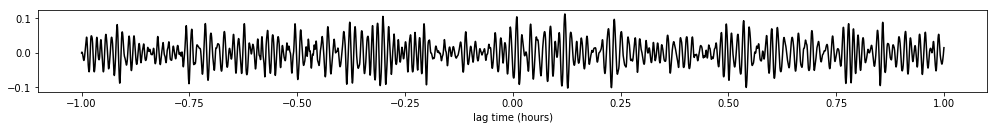Now comes the sugar. All cross-correlations are calculated and stacked in distance bins on the fly. We reuse the stacking function defined for the "real" arrivals. The processing takes 15 minutes on my PC. The stack is cached to a HDF5 file. A non-standard index using the inter-station distance has to be used, because traces in the stack do not have different seed ids.

In :
import obspyh5

obspyh5.set_index('{distance:06.2f}')

if os.path.exists(xstackfname):
print('Loaded file %s. To re-calculate cross-correlation stack delete this file.' % xstackfname)
else:
xcorrstack = stack_waveforms(xcorr_iter(pstream))
xcorrstack.write(xstackfname, 'H5')

Loaded file data/okhotsk_coda_xcorr_stack.h5. To re-calculate cross-correlation stack delete this file.


## Plot of the cross-correlation stack and results¶

Finally, the positive and negative side of the cross-correlation stack are added together and the stack is plotted like a travel time plot of "real" data. The top panel shows the number of traces stacked in the corresponding distance bin.

In :
# phase name: distance, lag time in minutes
PHASES = {'PcP': (50, 10),
'PKP': (150, 19),
'PKIKP': (181, 20),
'PP': (181, 26),
'PKPPcP': (181, 29),
'SKSP': (181, 37),
r'(PKP)$_2$': (60, 39),
r'(PKIKP)$_2$': (5, 40),
r'(PKP)$_2$PcP': (5, 49.5),
r'P$_4$': (-4, 53),
r'(PKP)$_2$(PcP)$_2$': (3, 58),
'ScS': (20, 16)}

def plot_xcorr_stack(stream, vmax=0.04):
stream.sort(['distance'])
dists = [tr.stats.distance for tr in stream]
nums = [tr.stats.num for tr in stream]
ddeg = dists - dists
fig = plt.figure(figsize=(10, 10))
ax1 = fig.add_axes([0.05, 0.86, 0.8, 0.08])
ax1.bar(dists, nums, ddeg, fc='#543005', alpha=0.5)
plt.setp(ax1.get_xticklabels(), visible=False)
ax1.set_ylabel('num pairs')
ax2 = fig.add_axes([0.05, 0.05, 0.8, 0.8], sharex=ax1)
imdata = []
for tr in stream:
N = len(tr)
imdata.append(0.5 * (tr.data[N // 2:] + tr.data[:N // 2 + 1][::-1]))
imdata = np.array(imdata).transpose()
sr = tr.stats.sampling_rate
extent = [dists - 0.5 * ddeg, dists[-1] + 0.5 * ddeg, -0.5 / sr / 60, 60 + 0.5 / sr / 60]
ax2.imshow(imdata, interpolation='nearest', aspect='auto', extent=extent,
origin='lower', vmin=-vmax, vmax=vmax, cmap='BrBG_r')
ax2.set_xlabel(u'inter-station distance (°)')
ax2.set_ylabel('lag time (minutes)')
ax2.set_yticks(10 * np.arange(6))
ax2.annotate(u'≈360,000 correlations', (1, 1), (-5, -5), 'axes fraction',
'offset points', va='top', ha='right', size='large')
for k, v in PHASES.items():
ax2.annotate(k, v, (0, 0), textcoords='offset points',
va='top', ha='left', annotation_clip=False)

plot_xcorr_stack(xcorrstack)
plt.show()